# Oberseminar Analysis Winter 2015/16

## Organizers: A. Arroyo-Rabasa, S. Conti, H. Koch, S. Müller, B. Niethammer, M. Rumpf, C. Thiele, J. López-Velázquez

• Thursday, October 15, 2:15 p.m., Lipschitz-Saal
Variational energies with a perimeter penalization and a Dirichlet part on a general class of gradients
We consider a class of set-valued energies that model certain physical problems such as the conductivity equations and other problems in linear and even non-linear elasticity. As part of the model, a regularizing term is added via a perimeter penalization. We prove partial regularity for minima in the sense of the theory of minimal surfaces and generalize those results from Ambrosio and Buttazzo, Lin and Lin and Kohn not only to the case of systems but to a more general class we call gradient type operators. Some examples and applications to problems in optimal design are further discussed.
• Thursday, November 5, 2:15 p.m. SR 0.011
Gianluca Orlando (SISSA, Trieste)
Cohesive fracture models for elasto-plastic materials as limit of gradient damage models coupled with plasticity
In this talk I will present a result about the asymptotic behavior of gradient damage models coupled with plasticity in the antiplane case. I will start with a brief overview of perfect plasticity and then I will explain how plasticity and damage are coupled. Next, I will state the main Gamma-convergence result: as the damage is forced to concentrate on hypersurfaces, these models behave as a model for cohesive fracture in elasto-plastic materials. I will show the proof of the result in the one dimensional case, and I will sketch the proof in the general case
• Thursday, November 12, 2:15 p.m., SR 0.011
Piotr Gwiazda (Polish Academy of Sciences, Warsaw)
On weak and measure-valued solutions to compressible Euler and similar systems
In recent years there has been an  important development in the theory
of incompressible Euler equations in multi-D. The important issues
that one could mention are the results on existence of weak solutions
by the method of convex integration and the results of strong  -
measure-valued uniqueness. In the talk I will concentrate on the
related results for various systems of compressible flows. I will
discuss two models:
• the shallow water kind model for granular avalanches
• Euler Alignment model for collective behaviour (similar to Euler-Poisson system).

• Thursday, November 19, 2:15 p.m., Lipschitz-Saal
Arthur Kierkels
On a kinetic equation arising in weak turbulence theory for the nonlinear Schrödinger equation
The vast majority of solutions to the (cubic) weak turbulence equation associated to the defocussing nonlinear Schrödinger equation converge in the sense of measures to a Dirac measure at zero. In this talk we start with the heuristic derivation of a quadratic equation that is thought to describe the long-time behaviour outside the origin of solutions with finite measure to the cubic one. It can be shown that for any nonnegative finite Radon measure there exists a weak solution. We will focus on two results. First we address the nonexistence of classical solutions, which we do by proving the instantaneous onset of a Dirac measure at zero for all weak solutions that have initial data that is nonzero on the positive real line. We then show existence of two different kinds of self-similar solutions; one with finite energy, and a one-parameter family of fat tailed solutions that have finite mass and infinite energy.
• Thursday, December 3, 2:15 p.m., SR 0.011
From absolutely to uniformly convergent fourier series:
a talk with two tales

The theory of absolutely convergent fourier series
is beautiful and well-developed, with many striking results.
In the 1950s, the schools of french and russian fourier
analysts were interested in exploring what happens when
one relaxes the condition of absolute convergence to
uniform convergence in various problems. We'll survey two
such examples -- (i) Sidon sets and (ii) the Beurling?Helson
theorem. In both cases, such explorations eventually made
connections with the Calderon-Zygmund school.

• Tuesday, March 1, 2:15 p.m., R0.007
Miroslav Bulicek (Charles University)
Nonlinear elliptic equations beyond the natural duality pairing:
Nonlinear elliptic system with given right hand side in divergence form $div f$ are one of the most studied systems. In case $f$ is good enough (i.e., sufficently integrable), one can solve such a problem by using the monotone operator theory, however in case $f$ is not sufficiently integrable,  no existence theory was available except the case when the leading elliptic operator is linear, e.g. the Laplace operator. Recently, several interesting new results have been obtained and we present an existence and uniqueness theory for certain class of nonlinear operators and $f$ not satisfying the natural duality pairing, which makes the theory identical to those for linear problems.

• Thursday, March 3, 10:00 a.m.,
Miroslav Bulicek (Charles University)
On the full up to the boundary regualrity  of minimizers to
variational integrals with linear growth on general nonconvex domain.

The problem of minimizing a functional F having the lineargrowth with respect to the gradient of the unknown is one of the oldestproblems. In certain cases, e.g. for minimal surface equation, it isknown that the minimizers are Lipschitz in the interior of the domain,however the prescribed trace is not attained. This is usually not the case on convex domains where all estimates can be done up to the boundary. In our work we identify a sharp class of strictly convex functionals for which one can establish regularity theory up to the boundary even for general non-convex domains, i.e. we provide a priori estimate on the gradient of the unknown up to the boundary, that further allows one to show smoothness of a minimizer in case of smooth data.

• Thursday, March 3, 11:00 a.m.,
Miroslav Bulicek (Charles University)
Pressure dependent viscosities in incompressible non-Newtonian
fluids - existence analysis
A generalization of Navier-Stokes' model is considered, where the Cauchy stress tensor depends on the pressure as well as on the shear rate in a power-law-like fashion, for values of the power-law index $r \in \bigl(\frac{2d}{d+2},2\bigr]$. We develop existence of generalized (weak) solutions for the resultant system of partial differential equations, including also the so far uncovered cases $r\in \bigl(\frac{2d}{d+2},\frac{2d+2}{d+2}\bigr]$ and  $r=2$. By considering a maximal sensible range of the power-law index $r$, the obtained theory is in effect identical to the situation of dependence on the shear rate only. The key difficulty here is to obatin the almost everywhere convergence of the pressure, which is essential for passing to the limit.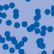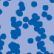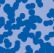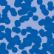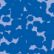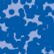## News

Florian Schweiger erhielt den Hausdorff-Gedächtnispreis 2021 der Fachgruppe Mathematik für die beste Dissertation. Er fertigte die Dissertation unter der Betreuung von Prof. Stefan Müller an. Unter anderen wurde Vanessa Ryborz mit einem Preis der Bonner Mathematischen Gesellschaft für ihre von Prof. Sergio Conti betreute Bachelorarbeit ausgezeichnet. (18.01.2022)

Prof. Dr. Sergio Albeverio has been elected into the Academia Europaea and the Accademia Nazionale dei Lincei (more; 02.12.2021).

Der SFB 1060 Die Mathematik der emergenten Effekte hat eine dritte Förderperiode erhalten. (26.11.20)

Prof. Dr. Andreas Eberle erhält den diesjährigen Lehrpreis der Universität Bonn. (22.07.2020)

Herr Dr. Richard Höfer erhielt den Hausdorff-Gedächtnispreis 2019 der Fachgruppe Mathematik für die beste Disseration. Betreut wurde die Arbeit von Prof. J. Velázquez (29.01.2020).

Contact

Managing Director: Prof. Dr. Massimiliano Gubinelli